Ex 7.3

Chapter 7 Class 6 Fractions
Serial order wise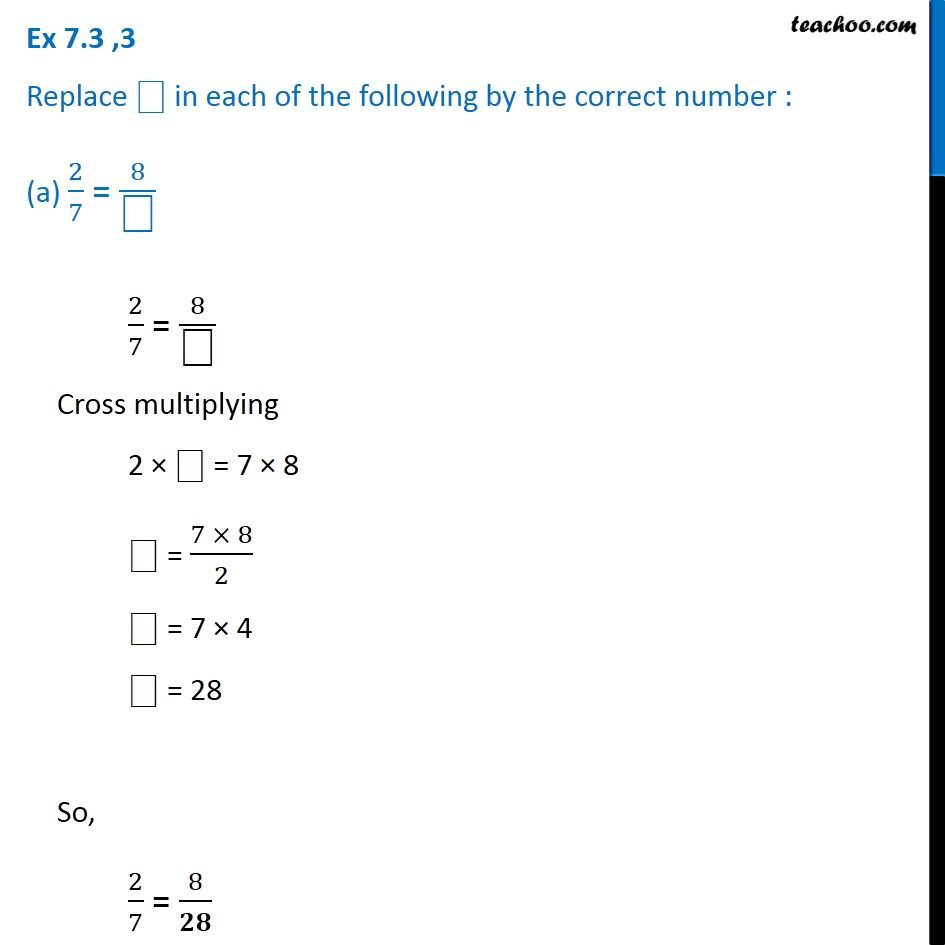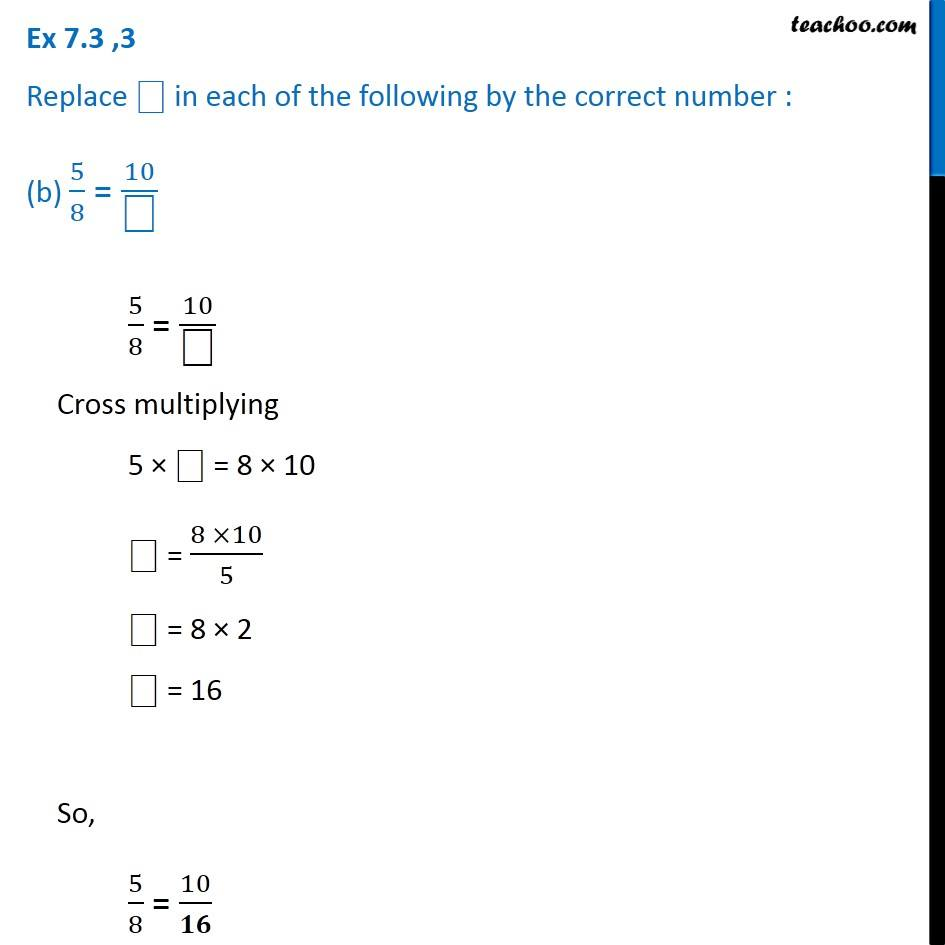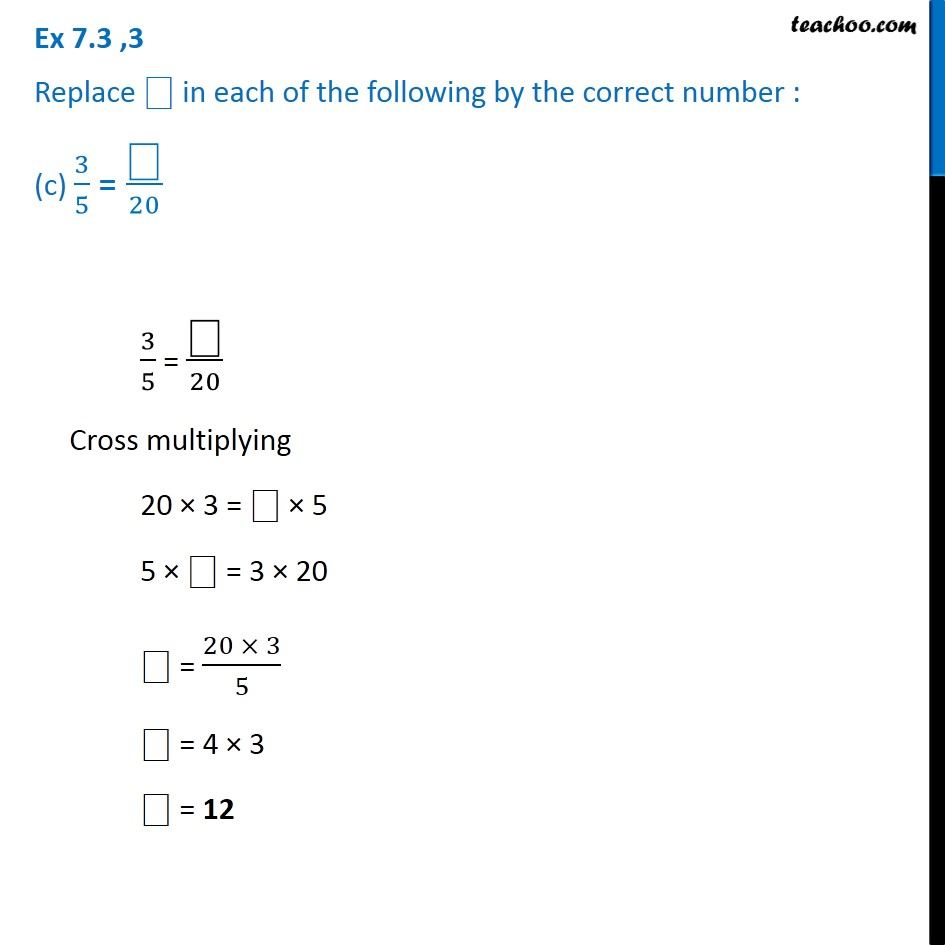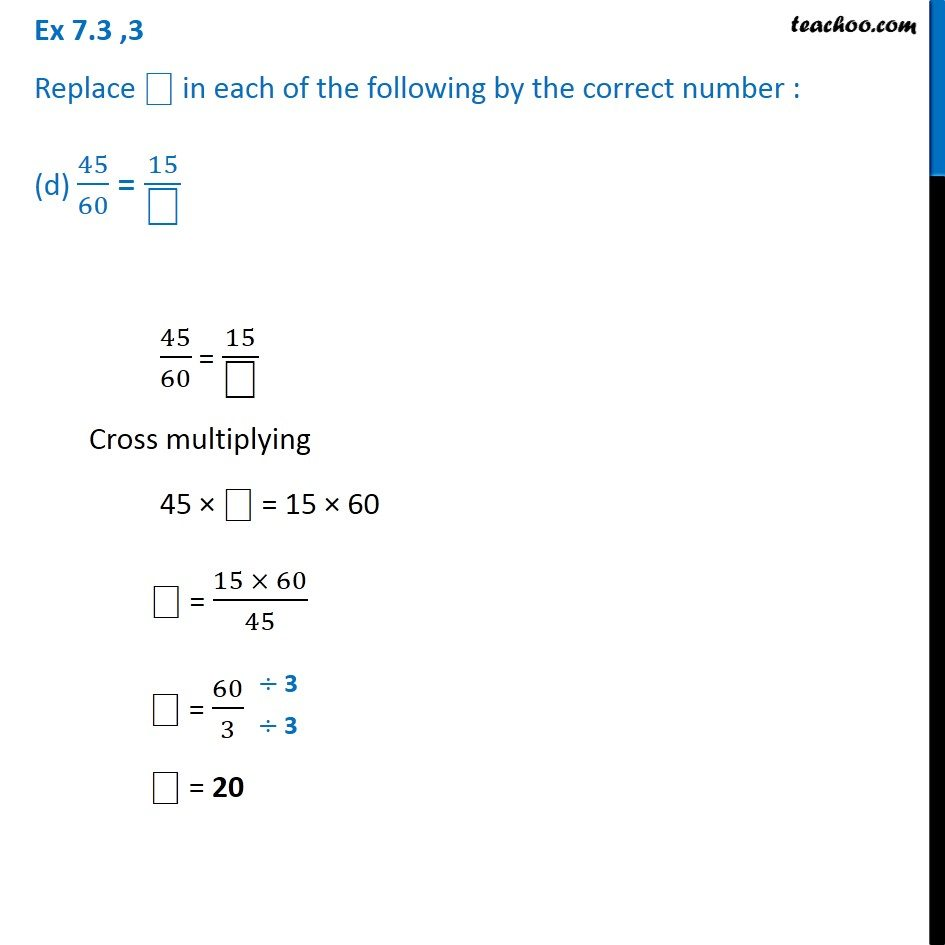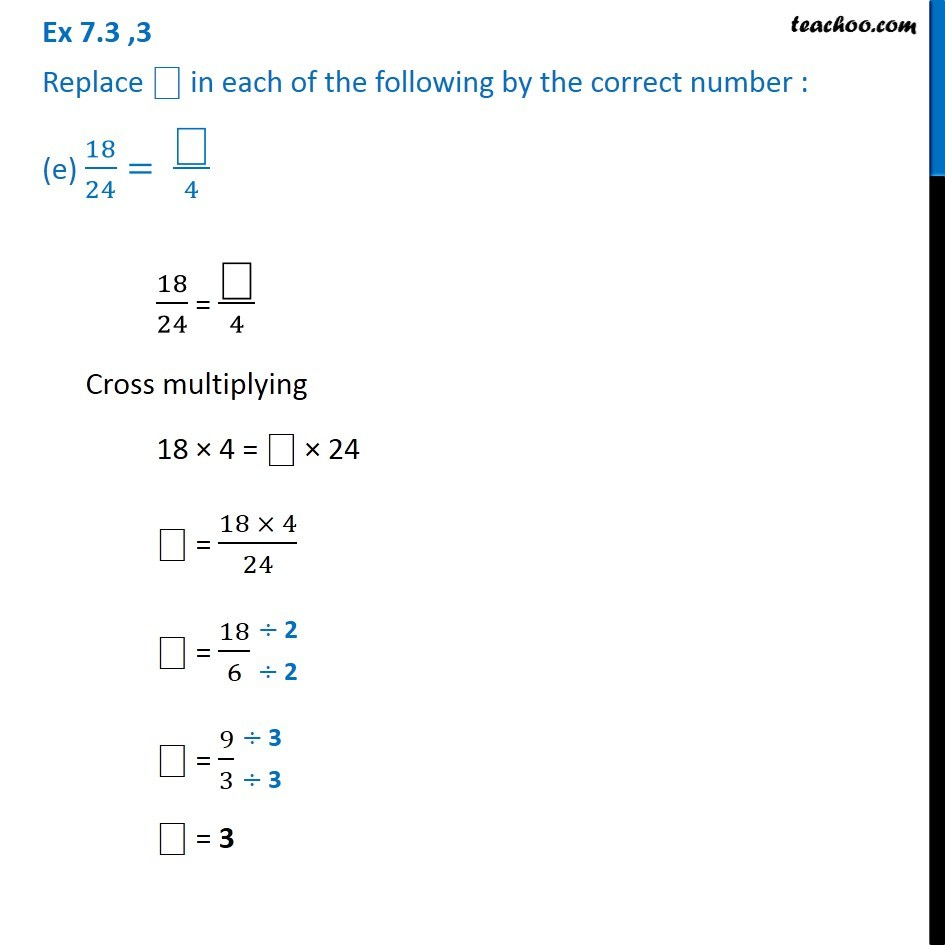Learn in your speed, with individual attention - Teachoo Maths 1-on-1 Class

### Transcript

Ex 7.3 ,3 Replace "⎕" in each of the following by the correct number : (a) 2/7 = 8/"⎕" 2/7 = 8/"⎕" Cross multiplying 2 × "⎕" = 7 × 8 "⎕" = (7 × 8)/2 "⎕" = 7 × 4 "⎕" = 28 So, 2/7 = 8/𝟐𝟖 Ex 7.3 ,3 Replace "⎕" in each of the following by the correct number : (b) 5/8 = 10/"⎕" 5/8 = 10/"⎕" Cross multiplying 5 × "⎕" = 8 × 10 "⎕" = (8 ×10)/5 "⎕" = 8 × 2 "⎕" = 16 So, 5/8 = 10/𝟏𝟔 Ex 7.3 ,3 Replace "⎕" in each of the following by the correct number : (c) 3/5 = "⎕" /20 3/5 = "⎕" /20 Cross multiplying 20 × 3 = "⎕" × 5 5 × "⎕" = 3 × 20 "⎕" = (20 × 3)/5 "⎕" = 4 × 3 "⎕" = 12 Ex 7.3 ,3 Replace "⎕" in each of the following by the correct number : (d) 45/60 = 15/"⎕" 45/60 = 15/"⎕" Cross multiplying 45 × ⎕ = 15 × 60 "⎕" = (15 × 60)/45 "⎕" = 60/3 "⎕" = 20 Ex 7.3 ,3 Replace "⎕" in each of the following by the correct number : (e) 18/24= "⎕" /4 18/24 = "⎕" /4 Cross multiplying 18 × 4 = "⎕" × 24 "⎕" = (18 × 4)/24 "⎕" = 18/6 "⎕" = 9/3 "⎕" = 3